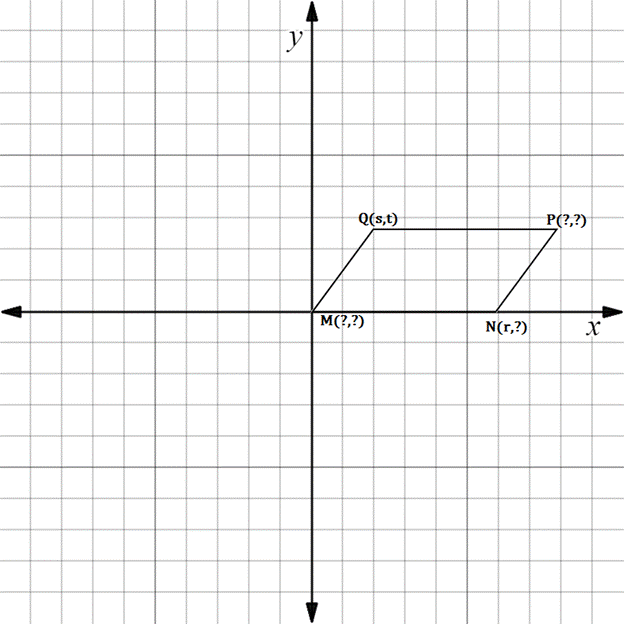Chapter 10.3, Problem 13E### Elementary Geometry for College St...

6th Edition
Daniel C. Alexander + 1 other
ISBN: 9781285195698

#### Solutions

Chapter
Section### Elementary Geometry for College St...

6th Edition
Daniel C. Alexander + 1 other
ISBN: 9781285195698
Textbook Problem
6 views

# In Exercises 11 to 16, supply the missing coordinates for the vertices, using as few variables as possible.M N P Q is a parallelogram.

To determine

To find:

The missing coordinates for the vertices.

Explanation

The given figure is shown below.

From the figure, the following observations are made.

Since, the vertex M is at the origin.

The coordinates of M0, 0.

Now, consider the vertex N.

Here, x-coordinate is given as r.

In the N vertex, observe where it lies on y-axis.

Since, it is at 0, the vertex N has the coordinates r, 0.

Consider the vertex P.

In the y-axis, it lies on the same line wher Q lies

### Still sussing out bartleby?

Check out a sample textbook solution.

See a sample solution

#### The Solution to Your Study Problems

Bartleby provides explanations to thousands of textbook problems written by our experts, many with advanced degrees!

Get Started

#### Find more solutions based on key concepts